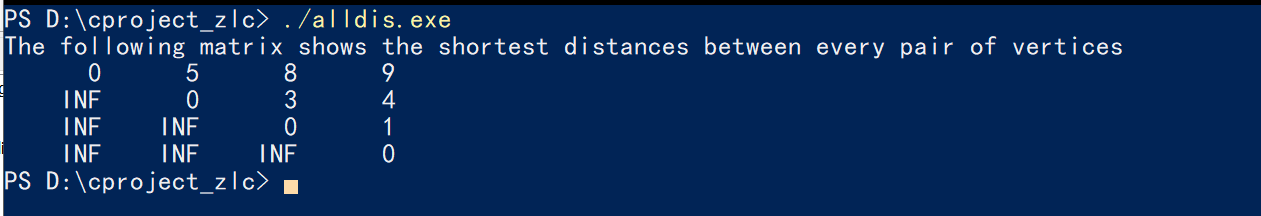# Question:Can Maple's functions be packaged as executable files?

## Question:Can Maple's functions be packaged as executable files?

Maple 2022

The problem comes from the link https://www.mapleprimes.com/questions/234398-Convert-Maple-Code-To-Python-#comment287424.

We know that when we compile a C or C ++ function, it generates an executable file.  Then we are free from source code.  For example. the function below returns a square matrix A where    "A[i, j]" is the distance from vertex i to vertex j in the graph G. My computer system is Windows.

```// C Program for Floyd Warshall Algorithm
#include <stdio.h>

// Number of vertices in the graph
#define V 4

/* Define Infinite as a large enough
value. This value will be used
for vertices not connected to each other */
#define INF 99999

// A function to print the solution matrix
void printSolution(int dist[][V]);

// Solves the all-pairs shortest path
// problem using Floyd Warshall algorithm
void floydWarshall (int graph[][V]) {
/* dist[][] will be the output matrix
that will finally have the shortest
distances between every pair of vertices */
int dist[V][V], i, j, k;

/* Initialize the solution matrix
same as input graph matrix. Or
we can say the initial values of
shortest distances are based
on shortest paths considering no
intermediate vertex. */
for (i = 0; i < V; i++)
for (j = 0; j < V; j++)
dist[i][j] = graph[i][j];

/* Add all vertices one by one to
the set of intermediate vertices.
---> Before start of an iteration, we
have shortest distances between all
pairs of vertices such that the shortest
distances consider only the
vertices in set {0, 1, 2, .. k-1} as
intermediate vertices.
----> After the end of an iteration,
vertex no. k is added to the set of
intermediate vertices and the set
becomes {0, 1, 2, .. k} */
for (k = 0; k < V; k++) {
// Pick all vertices as source one by one
for (i = 0; i < V; i++) {
// Pick all vertices as destination for the
// above picked source
for (j = 0; j < V; j++) {
// If vertex k is on the shortest path from
// i to j, then update the value of dist[i][j]
if (dist[i][k] + dist[k][j] < dist[i][j])
dist[i][j] = dist[i][k] + dist[k][j];
}
}
}

// Print the shortest distance matrix
printSolution(dist);
}

/* A utility function to print solution */
void printSolution(int dist[][V]) {
printf ("The following matrix shows the shortest distances"
" between every pair of vertices \n");
for (int i = 0; i < V; i++) {
for (int j = 0; j < V; j++) {
if (dist[i][j] == INF)
printf("%7s", "INF");
else
printf ("%7d", dist[i][j]);
}
printf("\n");
}
}

// driver program to test above function
int main() {
/* Let us create the following weighted graph
10
(0)------->(3)
|         /|\
5 |          |
|          | 1
\|/         |
(1)------->(2)
3           */
int graph[V][V] = { {0,   5,  INF, 10},
{INF, 0,   3, INF},
{INF, INF, 0,   1},
{INF, INF, INF, 0}
};

// Print the solution
floydWarshall(graph);
return 0;
}```

The above functions will be packaged as the disall.exe , and then we will move them anywhere in my computer and run it in Powershell.  We don't have to deal with the source code unless we want to change it.I mean can Maple do something like that?

```with(GraphTheory);
G := Graph([1, 2, 3, 4, 5], {{1, 2}, {1, 3}, {1, 4}, {1, 5}, {2, 3}, {2, 5}, {3, 4}, {4, 5}});
AllPairsDistance(G);
```

For exmaple, can I package the above code snippet into an exe file?﻿